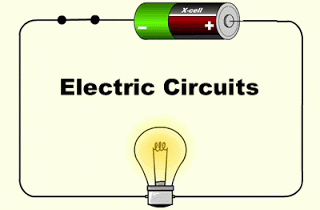# Circuits Basics

11 Questions | Total Attempts: 90SettingsStart here!

Related Topics
• 1.
What is current?
• A.

The flow of charges, usually in the form of electrons

• B.

The newness of a battery or resistor

• C.

Something that blocks or slows the flow of electrons

• D.

The potential energy stored in a battery

• 2.
What is the unit for current?
• A.

Volts

• B.

Amperes

• C.

Ohms

• D.

Joules

• 3.
Voltage is a measurement of what?
• A.

Radiant energy of a lightbulb in a circuit

• B.

Kinectic energy of a motor in a circuit

• C.

Potential energy stored in a battery

• D.

The flow of electrons

• 4.
What is the unit for voltage?
• A.

Volts

• B.

Amperes

• C.

Ohms

• D.

Joules

• 5.
What is the unit for resistance?
• A.

Volts

• B.

Amperes

• C.

Ohms

• D.

Joules

• 6.
What is the unit for energy?
• A.

Volts

• B.

Amperes

• C.

Ohms

• D.

Joules

• 7.
Which of these is the equation for Ohm's Law?
• A.

W = Fd

• B.

V = IR

• C.

R = IV

• D.

PE = mgh

• 8.
What is shown in this picture?
• A.

Resistors connected in parallel

• B.

Batteries connected in parallel

• C.

Resistors connected in series

• D.

Batteries connected in series

• 9.
Why can birds sit on a power line?
• A.

Because birds have minimal resistance compared to household electronics, so current is diverted to the houses instead

• B.

Because the wire is in the air and air has infinite resistance so no current flows through the wire

• C.

Because birds' feet are insulated so current is not conducted through them

• D.

Because both feet are on the same voltage line, so there is no potential difference, so current does not flow through the bird

• 10.
What if a bird sat on a power line that was very close to the ground, placing one foot on the wire and one foot on the ground?
• A.

No current would flow through the bird, so nothing would happen

• B.

The bird would experience a voltage difference between the wire and the ground and would get fried

• C.

The bird's feet are insulated, so it doesn't matter what it touches

• 11.
A certain steam iron carries a current of 6.4 A when connected to a 120 V source.  What is the resistance of the steam iron?
• A.

1.875 Ω

• B.

0.05 Ω

• C.

768 Ω

• D.

18.75 Ω